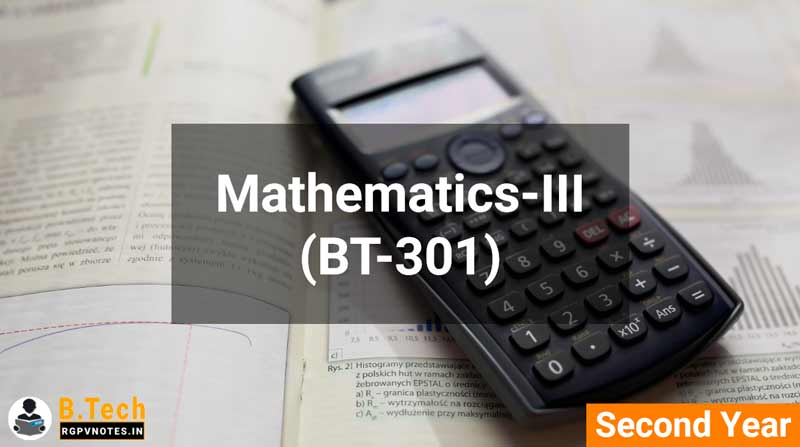# Mathematics-III (BT-301)## OBJECTIVES

OBJECTIVES: The objective of this course is to fulfill the needs of engineers to understand applications of Numerical Analysis, Transform Calculus and Statistical techniques in order to acquire mathematical knowledge and to solving wide range of practical problems appearing in different sections of science and engineering. More precisely, the objectives are:
 To introduce effective mathematical tools for the Numerical Solutions algebraic and transcendental equations.
 To enable young technocrats to acquire mathematical knowledge to understand Laplace transformation, Inverse Laplace transformation and Fourier Transform which are used in various branches of engineering.
 To acquaint the student with mathematical tools available in Statistics needed in various field of science and engineering

## Syllabus

UNIT 1:
Numerical Methods – 1: (8 hours): Solution of polynomial and transcendental equations – Bisection method, Newton-Raphson method and Regula-Falsi method. Finite differences, Relation between operators, Interpolation using Newton’s forward and backward difference formulae. Interpolation with unequal intervals: Newton’s divided difference and Lagrange’s formulae.

UNIT 2:
Numerical Methods – 2: (6 hours): Numerical Differentiation, Numerical integration: Trapezoidal rule and Simpson’s 1/3rd and 3/8 rules. Solution of Simultaneous Linear Algebraic Equations by Gauss’s Elimination, Gauss’s Jordan, Crout’s methods, Jacobi’s, Gauss-Seidal, and Relaxation method.,

UNIT 3:
Numerical Methods – 3: (10 hours): Ordinary differential equations: Taylor’s series, Euler and modified Euler’s methods. RungeKutta method of fourth order for solving first and second order equations. Milne’s and Adam’s predicator-corrector methods. Partial differential equations: Finite difference solution two dimensional Laplace equation and Poission equation, Implicit and explicit methods for one dimensional heat equation (Bender-Schmidt and CrankNicholson methods), Finite difference explicit method for wave equation.

UNIT 4:
Transform Calculus: (8 hours): Laplace Transform, Properties of Laplace Transform, Laplace transform of periodic functions. Finding inverse Laplace transform by different methods, convolution theorem. Evaluation of integrals by Laplace transform, solving ODEs by Laplace Transform method, Fourier transforms.

UNIT 5:
Concept of Probability: (8 hours): Probability Mass function, Probability Density Function, Discrete Distribution: Binomial, Poisson’s, Continuous Distribution: Normal Distribution, Exponential Distribution.

• Unit 1
• Unit 2
• Unit 3
• Unit 4
• Unit 5

## Books Recommended

Textbooks/References: 1. P. Kandasamy, K. Thilagavathy, K. Gunavathi, Numerical Methods, S. Chand & Company, 2nd Edition, Reprint 2012.
2. S.S. Sastry, Introductory methods of numerical analysis, PHI, 4th Edition, 2005.
3. Erwin kreyszig, Advanced Engineering Mathematics, 9th Edition, John Wiley & Sons, 2006.
4. B.S. Grewal, Higher Engineering Mathematics, Khanna Publishers, 35th Edition, 2010.
5. N.P. Bali and Manish Goyal, A text book of Engineering Mathematics, Laxmi Publications, Reprint, 2010.
6. Veerarajan T., Engineering Mathematics, Tata McGraw-Hill, New Delhi, 2008.
7. P. G. Hoel, S. C. Port and C. J. Stone, Introduction to Probability Theory, Universal Book Stall, 2003 (Reprint).
8. S. Ross, A First Course in Probability, 6th Ed., Pearson Education India, 2002.
9. W. Feller, An Introduction to Probability Theory and its Applications, Vol. 1, 3rd Ed., Wiley, 1968. Statistics

## You May Also Like

Contact author here.

#### Contact Us

###### Address :

Indore, Madhya Pradesh

###### Email :

[email protected]